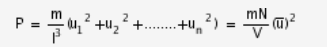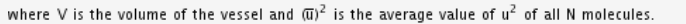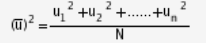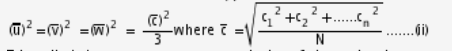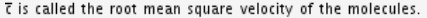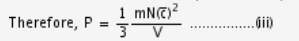# On the basis of the kinetic theory of gases derive an expression for the pressure

On the basis of the kinetic theory of gases derive an expression for the pressure exerted by a gas in terms of density and velocity of the molecules.

Consider a cubical vessel (of side l) with walls perfectly elastic as in the figure. Let the vessel have one gram molecule of a gas with its molecules in random motion.
Consider a molecule (mass m) moving with a velocity c, that can be resolved into three components u, v, and w in the direction of the edges of the cube along x, y, z ases.

Therefore, c2 = u2 + v2 + w2 …(i)

Let us consider two faces of the cube, say P and Q, normal to x-axis. When the molecule collides with the side Q with a velocity u, it rebounds with -u, while its other components remain unchanged.

Change in momentum of the molecule due to this collision = -2mu, which is imparted to the wall per collision.
The time taken by molecule to cover distance l = l/u
Therefore, after every interval of time 2l/u, the molecule will again collide with the wall Q, and the number of collisions per unit time with the wall Q is equal to u/2l.
Therefore, Momentum imparted to the wall per unit time = 2mu x u/2l = mu2/l (ignoring the negative sign).
The pressure exerted on the wall Q due to one molecule =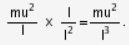If the vessel contains N molecules with velocities u1, u2, u3…un along x-axis, then the pressure exerted by them on Q, say